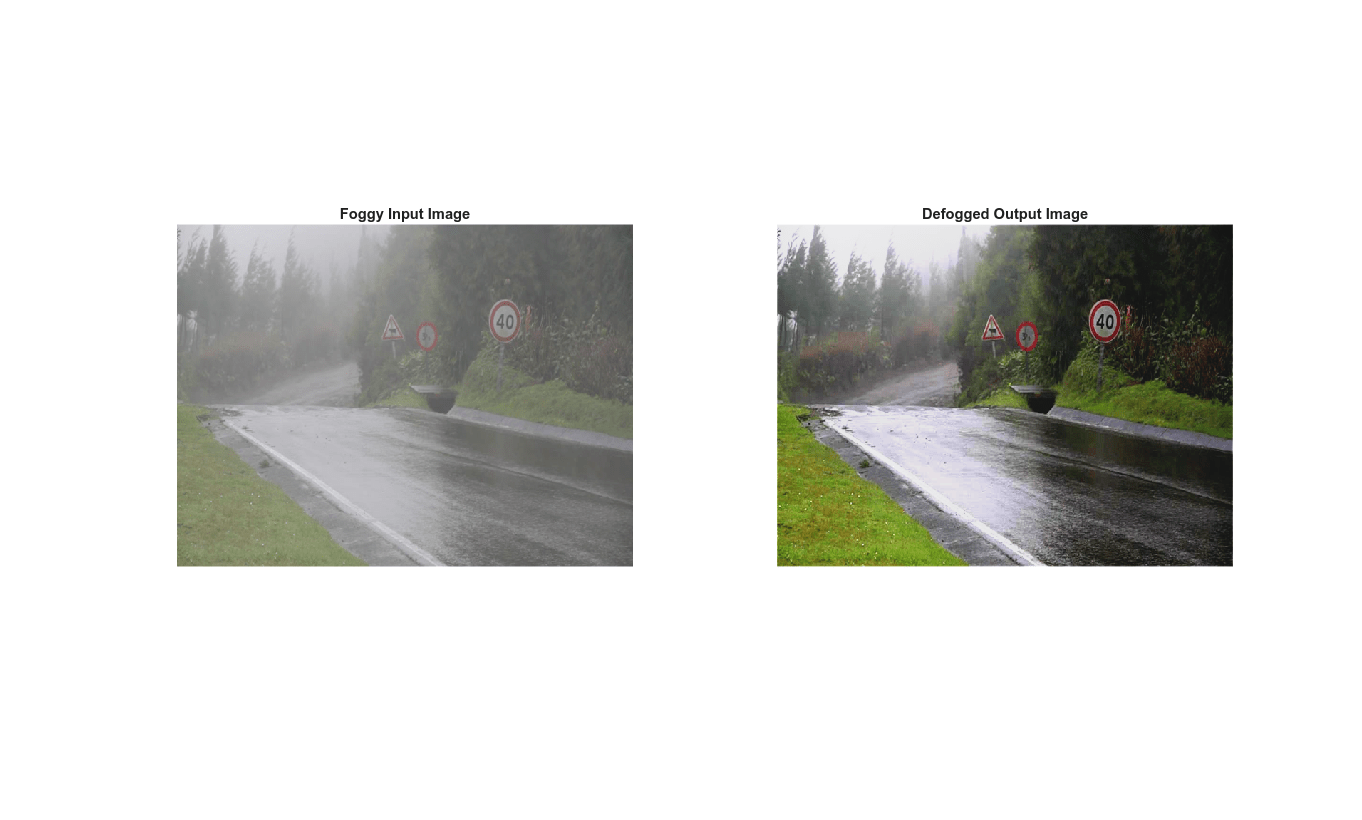# GPU Code Generation for a Fog Rectification Simulink Model

This example demonstrates how to generate CUDA® code from the Simulink® model that takes a foggy image as input and produces a defogged image as output. This example is a typical implementation of fog rectification algorithm. The example uses `conv2`, `im2gray`, and `imhist` (Image Processing Toolbox) functions. This example closely follows Fog Rectification example. This example illustrates the following concepts:

• Verification of GPU Environment.

• Model fog rectification application in Simulink by using image processing functions.

• Configure the model for GPU code generation.

• Generate a CUDA executable for the Simulink model.

### Third-Party Prerequisites

Required

This example generates CUDA MEX and has the following third-party requirements.

• CUDA enabled NVIDIA® GPU and compatible driver.

Optional

For non-MEX builds such as static, dynamic libraries or executables, this example has the following additional requirements.

### Verify GPU Environment

To verify that the compilers and libraries necessary for running this example are set up correctly, use the `coder.checkGpuInstall` function.

```envCfg = coder.gpuEnvConfig('host'); envCfg.BasicCodegen = 1; envCfg.Quiet = 1; coder.checkGpuInstall(envCfg); ```

The Simulink model for fog rectification consists of `Fog Rectification` subsystem that contains a `MATLAB Function` block which takes a foggy image as input and returns a defogged image as output. It uses `fog_rectification` algorithm described in Fog Rectification example. When the model runs, the `Visualization` block displays the foggy input image and defogged output image.

```mdl = 'fog_rectification_model'; open_system(mdl); ```### Configure Model for GPU Acceleration

Model configuration parameters determine the acceleration method used during simulation.

```set_param(mdl,'Solver','FixedStepAuto'); set_param(mdl,'GPUAcceleration','on'); set_param(mdl, 'SimulationMode','Normal'); ```

### Build GPU Accelerated Model

To build and simulate the GPU accelerated model, select Run on the Simulation tab or use the following MATLAB command:

```out = sim(mdl); ```### Configure Model for Code Generation

Set the following parameters for code generation.

```set_param(mdl,'TargetLang','C++'); set_param(mdl,'GenerateGPUCode','CUDA'); set_param(mdl,'GPUcuBLAS','on'); set_param(mdl,'GPUcuSOLVER','on'); set_param(mdl,'GPUcuFFT','on'); set_param(mdl,'ProdLongLongMode','on'); ```

### Generate CUDA Code for the Model

Generate and build the Simulink model on the host GPU by using the `slbuild` command. The code generator places the files in a build folder, a subfolder named `fog_rectification_model_ert_rtw` under your current working folder.

```status = evalc("slbuild('fog_rectification_model')"); ```

### Cleanup

```close_system('fog_rectification_model'); ```